Normal view

# Modern mathematical statistics with applications / Jay L Devore and Kenneth N Berk

Material type:TextLanguage: English Series: Springer texts in statisticsPublication details: ©2012. Edition: 2nd edDescription: xii, 845 p. : ill. ; 26 cmISBN: 9781461403906; 9781461403913Subject(s): Mathematical statistics | Statistique mathématiqueDDC classification: 311.2 Online resources: WorldCat details | E-book Fulltext
Contents:
TOC Overview and Descriptive Statistics -- Probability -- Discrete Random Variables and Probability Distributions -- Continuous Random Variables and Probability Distributions -- Joint Probability Distributions -- Statistics and Sampling Distributions -- Point Estimation -- Statistical Intervals Based on a Single Sample -- Tests of Hypotheses Based on a Single Sample -- Inferences Based on Two Samples -- The Analysis of Variance -- Regression and Correlation -- Goodness-of-Fit Tests and Categorical Data Analysis -- Alternative Approaches to Inference -- Appendix Tables.
Summary: Modern Mathematical Statistics with Applications, Second Edition strikes a balance between mathematical foundations and statistical practice. The text illustrates statistical concepts and methods through careful explanations and real-data applications.
Tags from this library: No tags from this library for this title.
Star ratingsAverage rating: 0.0 (0 votes)
Holdings
Item type Current library Collection Call number Copy number Status Date due Barcode Item holdsE-Book
E-book
Non-fiction 311.2 DEM 2012 (Browse shelf(Opens below)) Not for loanText
Reserve Section
Non-fiction 311.2 DEM 2012 (Browse shelf(Opens below)) C-1 Not For Loan 28194
Total holds: 0
##### Browsing EWU Library shelves, Shelving location: Reserve Section Close shelf browser (Hides shelf browser)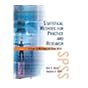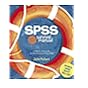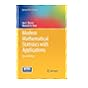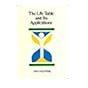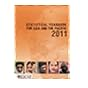309.1095492 INC 2013 Inclusive Finance and Sustainable Development: 310.072 GAS Statistical methods for practice and research : 310.072 PAS 2005 SPSS survival manual : 311.2 DEM 2012 Modern mathematical statistics with applications / 312.2 CHL 1984 The life table and its applications / 312.2 CHL 1984 The life table and its applications / 315 STA 2011 Statistical Yearbook for Asia and the Pacific 2011

Includes indexes.

TOC Overview and Descriptive Statistics --
Probability --
Discrete Random Variables and Probability Distributions --
Continuous Random Variables and Probability Distributions --
Joint Probability Distributions --
Statistics and Sampling Distributions --
Point Estimation --
Statistical Intervals Based on a Single Sample --
Tests of Hypotheses Based on a Single Sample --
Inferences Based on Two Samples --
The Analysis of Variance --
Regression and Correlation --
Goodness-of-Fit Tests and Categorical Data Analysis --
Alternative Approaches to Inference --
Appendix Tables.

Modern Mathematical Statistics with Applications, Second Edition strikes a balance between mathematical foundations and statistical practice. The text illustrates statistical concepts and methods through careful explanations and real-data applications.

AS

Sagar Shahanawaz

There are no comments on this title.